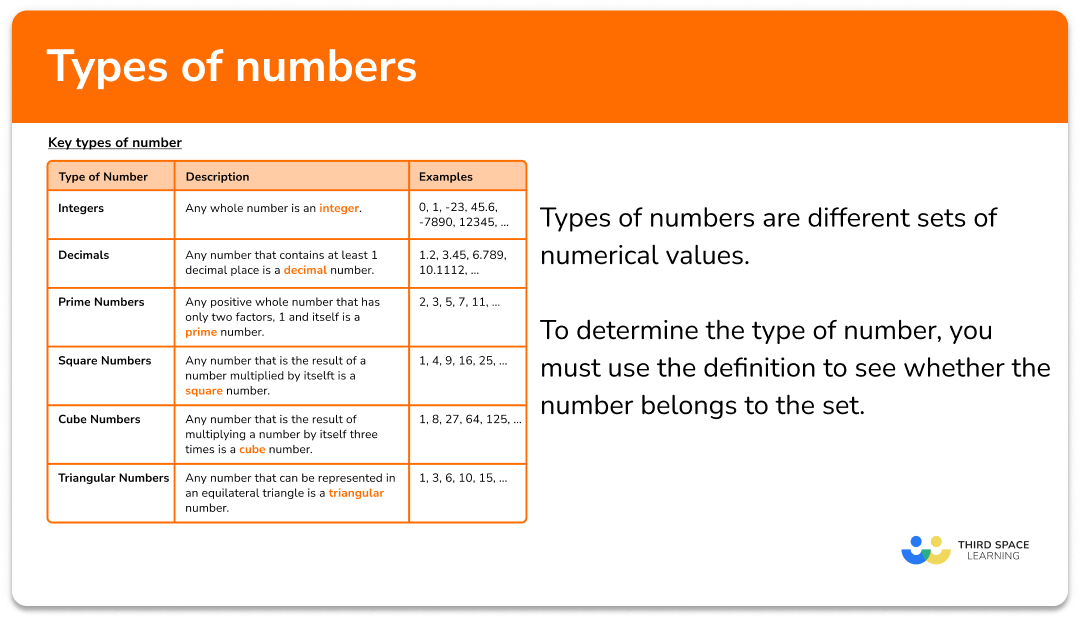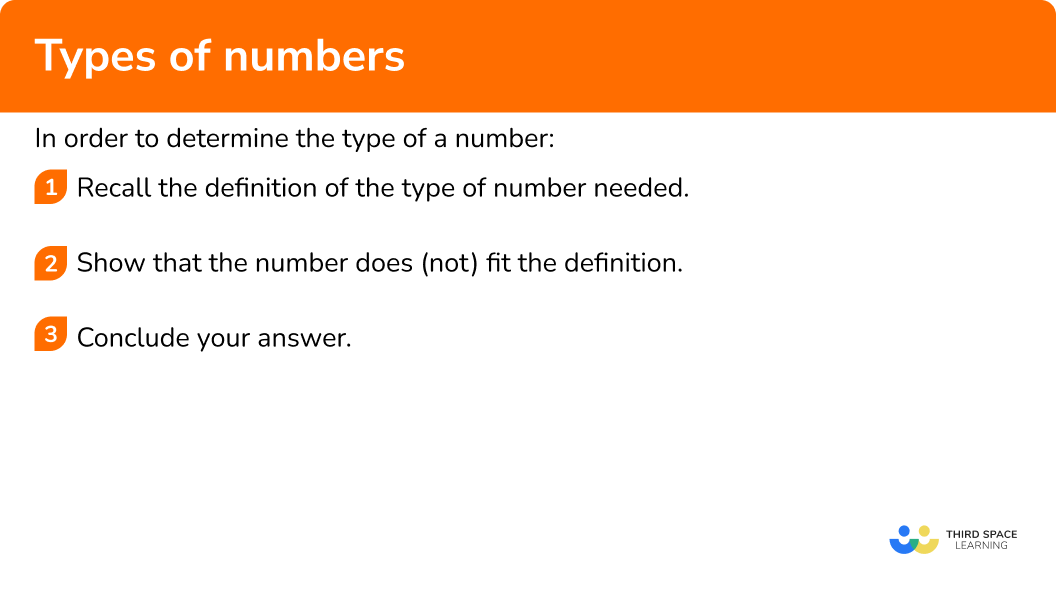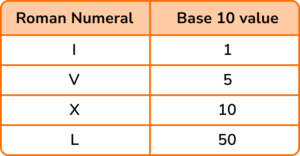GCSE Maths Number

Types Of Numbers

# Types Of Numbers

Here we will learn about different types of numbers and how to solve problems using them.

There are also types of numbers worksheets based on Edexcel, AQA and OCR exam questions, along with further guidance on where to go next if you’re still stuck.

## What are types of numbers?

Types of numbers are different sets of numerical values.

To determine the type of number, you must use the definition to see whether the number belongs to the set.

### Understand number definitions and terms

A number is a count or measurement.

We write or talk about numbers using numerals such as ‘6’. We need to know the different types of numbers to be able to solve problems.

• Positive and negative numbers

Any number above zero is a positive number and any number below zero is a negative number.

• Real numbers

All numbers on a number line are real numbers.

Real numbers can also be classified as rational or irrational.

• Rational and irrational numbers

We can make a rational number by dividing an integer by an integer.

Irrational numbers are the real numbers that cannot be expressed in fraction form.

Step-by-step guide: Rational numbers

• Natural numbers are positive integers (including 0 ). We can also call these ‘counting’ numbers’.

For example,

• Even and odd

Even numbers are divisible by 2 without remainders; they end in 0, 2, 4, 6 or 8.

Odd numbers are not divisible by 2 without remainders and end in 1, 3, 5, 7 or 9.

For example,

• Decimals

We can recognise decimal numbers because they have a whole number and a fractional part. The decimal point separates the whole number from the fractional part.

For example,

Step-by-step guide: Decimals

• Prime numbers and composite numbers

A prime number is a number which is only divisible by 1 and itself. We can define composite numbers as whole numbers that have more than two factors.

For example,

3 = 3 \times 1, so 3 is prime.

6= 3 \times 2, or 6= 1 \times 6. \ 6 has more than two factors, so 6 is composite.

We also need to recognise the properties of some other ‘special’ numbers

Step-by-step guide: Prime numbers

• Square numbers

Square numbers are the product of a number that has been multiplied by itself.

We can show square numbers using a pattern of dots in a square.

For example,

Step-by-step guide: Squares and square roots

• Cube numbers

A Cube number is the product when a number has been multiplied by itself and then itself again. We can show cube numbers using 3D cubes.

For example,

Step-by-step guide: Cube and cube roots

• Triangular numbers

A Triangular number is a number that can be shown using a pattern of dots in an equilateral triangle. We can find a triangular number by cumulatively adding natural numbers.

For example,

Step-by-step guide: Triangular numbers

• Reciprocal maths

A reciprocal of a number is its multiplicative inverse. This means that if you multiply a number by its reciprocal the answer is 1.

To find a reciprocal you divide 1 by the number.

For example, the reciprocal of 3 is \frac{1}{3}.

Step-by-step guide: Reciprocal maths (coming soon)

### What are types of numbers?### Key types of number

Below is a description of each of these types of numbers, along with a few examples.

### Fractions and decimals

There are also a few specific types of numbers involving fractions and decimals that you are expected to understand. Below is a table outlining these different types of fractions and decimals.

## How to determine the type of a number

In order to determine the type of a number:

1. Recall the definition of the type of number needed.
2. Show that the number does (not) fit the definition.

### Explain how to determine the type of a number## Types of number examples

### Example 1: square numbers

Show that the number 36 is a square number.

1. Recall the definition of the type of number needed.

The definition of a square number is any number that is the result of a number multiplied by itself.

2Show that the number does (not) fit the definition.

36=6 \times 6

36 is a square number because it can be calculated by multiplying 6 by itself.

### Example 2: prime numbers

Determine whether the number 23 is a prime number.

The definition of a prime number is any positive whole number that has only two factors, 1 and itself.

The factors of 23 are 1 and 23.

23 is a prime number as it only has two factors, 1 and 23 (itself).

### Example 3: rational numbers

Show that \sqrt{3}\times\sqrt{27} is a rational number.

The definition of a rational number is any number that can be expressed as a fraction where the numerator and denominator are integers.

Completing the calculation, we have

\sqrt{3}\times\sqrt{27}=\sqrt{81}=9.

\sqrt{3}\times\sqrt{27}=9, which is a rational number as 9 can be written as a fraction where the numerator and denominator are integers, \frac{9}{1}.

Step-by-step guide: Surds

### Example 4: recurring decimal

Show that \frac{2}{9} is a recurring decimal.

The definition of a recurring decimal is any decimal where the decimal places repeat indefinitely.

We need to calculate 2 \div 9 to show that the decimal repeats itself. Using long division, we have

This means that \frac{2}{9}=0.22…=0.\dot{2}.

\frac{2}{9} =0.\dot{2}, which is a recurring decimal.

Step-by-step guide: Recurring decimals to fractions

### Example 5: mixed number

Write \frac{21}{4} as a mixed number.

The definition of a mixed number is any fraction that has a whole number part and a fractional part. The fractional part is a proper fraction.

Currently we have an improper fraction. To convert this to a mixed number, we need to calculate how many times the denominator goes into the numerator and determine the remainder.

21\div{4}=5 remainder 1, and so the whole number part of the mixed number is 5.

The fractional part of the mixed number has a numerator of 1, and as we are dividing by 4, the denominator stays as 4.

So we have \frac{21}{4}=5\frac{1}{4}.

\frac{21}{4}=5\frac{1}{4} as a mixed number.

### Example 6: cube number in index form

The number 592704=2^{6}\times{3}^{3}\times{7}^{3}. Show that 592704 is a cube number.

The definition of a cube number is any number that is the result of multiplying a number by itself three times.

Using laws of indices, the cube root of 592704 is the same as

\begin{aligned} 592704&=(2^{6}\times{3}^{3}\times{7}^{3})^{\frac{1}{3}}\\\\ &=2^{\frac{6}{3}}\times{3}^{\frac{3}{3}}\times{7}^{\frac{3}{3}}\\\\ &=2^{2}\times{3}\times{7}\\\\ &=4\times{3}\times{7}\\\\ &=84 \end{aligned}

The cube root of 592704 is 84, so 592704 is a cube number (84^{3}).

### Common misconceptions

• One type of number is another (mixed up definitions)

It is very common that definitions get mixed up, for example, a recurring decimal is the opposite of a terminating decimal. The set of numbers that are not terminating decimals are non-terminating decimals. Within that set is the subset of recurring decimals.

All recurring decimals are non-terminating, but not all non-terminating decimals are recurring (think of \pi and \frac{1}{9} ).

• Categorising zero as a natural number

Zero is not a natural number because these numbers are used as counting numbers and so the first natural number is 1.

• Mixing up squares, cubes and their roots

The square root of a number is written as the value squared, for example, \sqrt{9}=3^2 which is incorrect. The square root of 9 is 3 because 3 \times 3=9.

• Not recognising whole numbers as rational numbers

Rational numbers are numbers that can be expressed as a fraction where the numerator and the denominator are integers. The number 3 is rational because we can express 3 as the fraction \frac{3}{1}.

• Fractions cannot contain decimals

They can, they just look mean! Take the fraction \frac{2.3}{4.6}. At first glance, this does not look pleasant but by using equivalent fractions, we can multiply both the numerator and denominator by 10 to get \frac{23}{46}.

A common factor of both of these numbers is 23 and so this is equivalent to \frac{1}{2}. When we simplify fractions, we deliberately avoid decimals, because the fractions become more complicated.

### Practice types of numbers questions

1. What type of number is 22?

Natural NumberRecurring DecimalProper FractionComplex Number22 is a natural number because any number that is used as a counting number is a natural number.

2. What type of number is 2.43?

Composite NumberMixed NumberImaginary NumberTerminating Decimal2.43 is a terminating decimal because any decimal with a finite number of decimal places is a terminating decimal.

3. What type of number is \frac{\sqrt{2}}{5}?

Improper FractionPerfect NumberIrrational NumberExponent\frac{\sqrt{2}}{5} is an irrational number because the numerator is not an integer and the definition of an irrational number is any number that cannot be expressed as a fraction where the numerator and denominator are both integers.

4. What type of number is 2^{8}\times{3}^{6}\times{5}^{12}?

Triangular NumberCube NumberSquare NumberOrdinary Number2^{8}\times{3}^{6}\times{5}^{12} is a square number because all of the exponents are even.

\sqrt{2^{8}\times{3}^{6}\times{5}^{12}}=(2^{8}\times{3}^{6}\times{5}^{12})^{\frac{1}{2}}=2^{4}\times{3}^{3}\times{5}^{6}

5. What type of number is 28?

Ordinary NumberPerfect NumberPrime NumberTranscendental NumberThe factors of 28 are, 1, 2, 4, 7, 14, and 28.

1+2+4+7+14=28 and so 28 is a perfect number.

6. What type of number is \frac{\sqrt{25}\times\sqrt{4}}{0.025}?

Complex NumberIrrational NumberNon-terminating DecimalSquare NumberCompleting the calculation we have

\frac{\sqrt{25}\times\sqrt{4}}{0.025}=\frac{\sqrt{100}}{0.025}=\frac{10}{0.025}=400.

400=20\times{20} and so \frac{\sqrt{25}\times\sqrt{4}}{0.025} is a square number.

### Types of number GCSE questions

1. Below is a table showing the base 10 values for some roman numerals.(a) Given that 23 is written as XXIII, and 49 is written as IL, what is the value of 49-23 in roman numerals?

(b) What type of number is your answer to part (a) in base 10? Circle all that may apply.

Positive Integer Terminating Decimal

Rational NumberNatural NumberPrime Number

(3 marks)

(a)

49-23=26 which is written as XXVI .

(1)

(b)

Any two of the following: Positive Integer, Rational Number, Natural Number.

(1)

All three.

(1)

2. Below is a list of five numbers.

(a) Which number listed above is an irrational number?

(b) List all of the numbers above that are recurring decimals.

(2 marks)

(a)

\frac{\pi}{2}

(1)

(b)

3.\dot{3} and \frac{2}{7} .

(1)

3. (a) Show that \sqrt{2}(\sqrt{2} \times \sqrt{4}) is a square number.

(b) Show that \frac{3}{8} \times 16 is a perfect number.

(5 marks)

(a)

\sqrt{2}(\sqrt{2} \times 2) or \sqrt{2} \times 2\sqrt{2}

(1)

2 \times \sqrt{4}=2 \times 2=4

(1)

(b)

\frac{3}{8} \times 16=3 \times 2=6

(1)

Factors of 6: 1, 2, 3, 6

(1)

1+2+3=6

(1)

## Learning checklist

You have now learned how to:

• Understand and use the vocabulary of different types of numbers
• Solve problems by reasoning using types of numbers

## Beyond GCSE

For GCSE Mathematics, the discriminant of the quadratic formula b^{2}-4ac has 3 possible conditions that determine the number and type of roots of a quadratic equation of the form ax^{2}+bx+c=0. We say that for

• b^{2}-4ac>0, we have two distinct, real roots
• b^{2}-4ac=0, we have one repeated, real root
• b^{2}-4ac<0, there are no real solutions.

For the third type b^{2}-4ac<0, the solutions are complex numbers. This is covered in detail at A Level.

## Still stuck?

Prepare your KS4 students for maths GCSEs success with Third Space Learning. Weekly online one to one GCSE maths revision lessons delivered by expert maths tutors.

Find out more about our GCSE maths tuition programme.